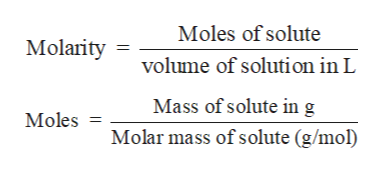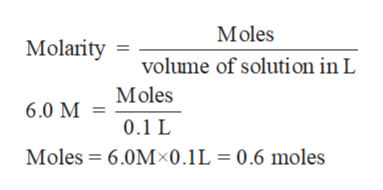# What volume of carbon dioxide gas at 0.980 atm and 293 K could be made from reacting 10.0 grams of sodium carbonate with 100. mL of 6.0 M HCl? This is a limiting reactant problem.Given: Na2CO3 (s) + 2 HCl (aq) -> 2 NaCl (aq) + H2O (g) + CO2 (g)

Question
1 views

What volume of carbon dioxide gas at 0.980 atm and 293 K could be made from reacting 10.0 grams of sodium carbonate with 100. mL of 6.0 M HCl? This is a limiting reactant problem.
Given: Na2CO3 (s) + 2 HCl (aq) -> 2 NaCl (aq) + H2O (g) + CO2 (g)

check_circle

Step 1

Solution stoichiometry is mainly based on the calculation of moles and volumes. These two values are used to calculate the molarity of solution. The relation between moles, volume and molarity is as given below;help_outlineImage TranscriptioncloseMoles of solute Molarity volume of solution in L Mass of solute ing Molar mass of solute (g/mol) Moles = fullscreen
Step 2

Calculate moles of Na2CO3:

Molar mass of Na2CO3 = 106 g/mol

Mass of Na2CO3 = 10.0 g

Step 3

Calculate moles of HCl:

Volume of HCl = 100 mL  =...help_outlineImage TranscriptioncloseMoles Molarity volume of solution in L Moles 6.0 M 0.1 L Moles 6.0M-0.1L = 0.6 moles fullscreen

### Want to see the full answer?

See Solution

#### Want to see this answer and more?

Solutions are written by subject experts who are available 24/7. Questions are typically answered within 1 hour.*

See Solution
*Response times may vary by subject and question.
Tagged in

### Ideal gases